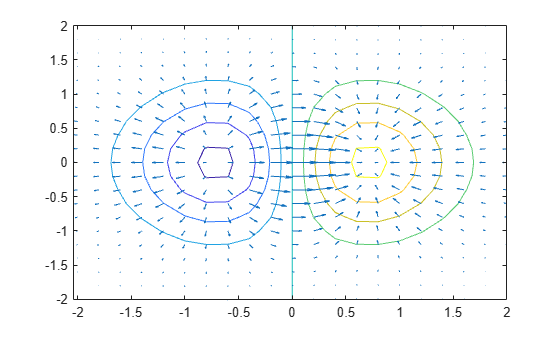## Syntax

``FX = gradient(F)``
``````[FX,FY] = gradient(F)``````
``````[FX,FY,FZ,...,FN] = gradient(F)``````
``[___] = gradient(F,h)``
``[___] = gradient(F,hx,hy,...,hN)``

## Description

example

````FX = gradient(F)` returns the one-dimensional numerical gradient of vector `F`. The output `FX` corresponds to ∂F/∂x, which are the differences in the x (horizontal) direction. The spacing between points is assumed to be `1`.```

example

``````[FX,FY] = gradient(F)``` returns the x and y components of the two-dimensional numerical gradient of matrix `F`. The additional output `FY` corresponds to ∂F/∂y, which are the differences in the y (vertical) direction. The spacing between points in each direction is assumed to be `1`.```
``````[FX,FY,FZ,...,FN] = gradient(F)``` returns the `N` components of the numerical gradient of `F`, where `F` is an array with `N` dimensions.```

example

````[___] = gradient(F,h)` uses `h` as a uniform spacing between points in each direction. You can specify any of the output arguments in previous syntaxes.```

example

````[___] = gradient(F,hx,hy,...,hN)` specifies `N` spacing parameters for the spacing in each dimension of `F`.```

## Examples

collapse all

Calculate the gradient of a monotonically increasing vector.

`x = 1:10`
```x = 1×10 1 2 3 4 5 6 7 8 9 10 ```
`fx = gradient(x)`
```fx = 1×10 1 1 1 1 1 1 1 1 1 1 ```

Calculate the 2-D gradient of $x{e}^{-{x}^{2}-{y}^{2}}$ on a grid.

```x = -2:0.2:2; y = x'; z = x .* exp(-x.^2 - y.^2); [px,py] = gradient(z);```

Plot the contour lines and vectors in the same figure.

```figure contour(x,y,z) hold on quiver(x,y,px,py) hold off```Use the gradient at a particular point to linearly approximate the function value at a nearby point and compare it to the actual value.

The equation for linear approximation of a function value is

`$f\left(x\right)\approx f\left({x}_{0}\right)+{\left(\nabla f\right)}_{{x}_{0}}\cdot \left(x-{x}_{0}\right).$`

That is, if you know the value of a function $f\left({x}_{0}\right)$ and the slope of the derivative ${\left(\nabla f\right)}_{{x}_{0}}$ at a particular point ${x}_{0}$, then you can use this information to approximate the value of the function at a nearby point $f\left(x\right)=f\left({x}_{0}+ϵ\right)$.

Calculate some values of the sine function between -1 and 0.5. Then calculate the gradient.

```y = sin(-1:0.25:0.5); yp = gradient(y,0.25);```

Use the function value and derivative at `x = 0.5` to predict the value of `sin(0.5005)`.

`y_guess = y(end) + yp(end)*(0.5005 - 0.5)`
```y_guess = 0.4799 ```

Compute the actual value for comparison.

`y_actual = sin(0.5005)`
```y_actual = 0.4799 ```

Find the value of the gradient of a multivariate function at a specified point.

Consider the multivariate function $f\left(x,y\right)={x}^{2}{y}^{3}$.

```x = -3:0.2:3; y = x'; f = x.^2 .* y.^3; surf(x,y,f) xlabel('x') ylabel('y') zlabel('z')```Calculate the gradient on the grid.

`[fx,fy] = gradient(f,0.2);`

Extract the value of the gradient at the point `(1,-2)`. To do this, first obtain the indices of the point you want to work with. Then, use the indices to extract the corresponding gradient values from `fx` and `fy`.

```x0 = 1; y0 = -2; t = (x == x0) & (y == y0); indt = find(t); f_grad = [fx(indt) fy(indt)]```
```f_grad = 1×2 -16.0000 12.0400 ```

The exact value of the gradient of $f\left(x,y\right)={x}^{2}{y}^{3}$ at the point (1,-2) is

`$\begin{array}{cl}{\left(\nabla f\right)}_{\left(1,-2\right)}& =2x{y}^{3}\underset{}{\overset{ˆ}{i}}+3{x}^{2}{y}^{2}\underset{}{\overset{ˆ}{j}}\\ & =-16\underset{}{\overset{ˆ}{i}}+12\underset{}{\overset{ˆ}{j}}.\end{array}$`

## Input Arguments

collapse all

Input array, specified as a vector, matrix, or multidimensional array.

Data Types: `single` | `double`
Complex Number Support: Yes

Uniform spacing between points in all directions, specified as a scalar.

Example: `[FX,FY] = gradient(F,2)`

Data Types: `single` | `double`
Complex Number Support: Yes

Spacing between points in each direction, specified as separate inputs of scalars or vectors. The number of inputs must match the number of array dimensions of `F`. Each input can be a scalar or vector:

• A scalar specifies a constant spacing in that dimension.

• A vector specifies the coordinates of the values along the corresponding dimension of `F`. In this case, the length of the vector must match the size of the corresponding dimension.

Example: `[FX,FY] = gradient(F,0.1,2)`

Example: `[FX,FY] = gradient(F,[0.1 0.3 0.5],2)`

Example: `[FX,FY] = gradient(F,[0.1 0.3 0.5],[2 3 5])`

Data Types: `single` | `double`
Complex Number Support: Yes

## Output Arguments

collapse all

Numerical gradients, returned as arrays of the same size as `F`. The first output `FX` is always the gradient along the 2nd dimension of `F`, going across columns. The second output `FY` is always the gradient along the 1st dimension of `F`, going across rows. For the third output `FZ` and the outputs that follow, the `N`th output is the gradient along the `N`th dimension of `F`.

collapse all

The numerical gradient of a function is a way to estimate the values of the partial derivatives in each dimension using the known values of the function at certain points.

For a function of two variables, F(x,y), the gradient is

`$\nabla F=\frac{\partial F}{\partial x}\stackrel{^}{i}+\frac{\partial F}{\partial y}\stackrel{^}{j}\text{\hspace{0.17em}}.$`

The gradient can be thought of as a collection of vectors pointing in the direction of increasing values of F. In MATLAB®, you can compute numerical gradients for functions with any number of variables. For a function of N variables, F(x,y,z, ...), the gradient is

`$\nabla F=\frac{\partial F}{\partial x}\stackrel{^}{i}+\frac{\partial F}{\partial y}\stackrel{^}{j}+\frac{\partial F}{\partial z}\stackrel{^}{k}+...+\frac{\partial F}{\partial N}\stackrel{^}{n}\text{\hspace{0.17em}}.$`

## Tips

• Use `diff` or a custom algorithm to compute multiple numerical derivatives, rather than calling `gradient` multiple times.

## Algorithms

`gradient` calculates the central difference for interior data points. For example, consider a matrix with unit-spaced data, `A`, that has horizontal gradient `G = gradient(A)`. The interior gradient values, `G(:,j)`, are

`G(:,j) = 0.5*(A(:,j+1) - A(:,j-1));`

The subscript `j` varies between `2` and `N-1`, with `N = size(A,2)`.

`gradient` calculates values along the edges of the matrix with single-sided differences:

```G(:,1) = A(:,2) - A(:,1); G(:,N) = A(:,N) - A(:,N-1);```

If you specify the point spacing, then `gradient` scales the differences appropriately. If you specify two or more outputs, then the function also calculates differences along other dimensions in a similar manner. Unlike the `diff` function, `gradient` returns an array with the same number of elements as the input.